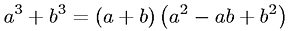Algebra > PolynomialsShowing results 1 to 7 of 7, on page 1 of 1
 DESCRIPTION EQUATION Difference of Cubes Factorization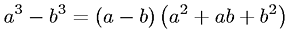Difference of Squares FactorizationPolynomial FOIL operation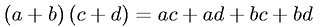Quadratic Formula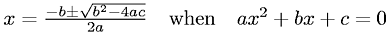Second Order Polynomial FactorizationSquare of a First Order Polynomial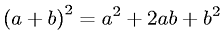Sum of Cubes Factorization